Related Articles

# Class 9 NCERT Solutions- Chapter 13 Surface Areas And Volumes – Exercise 13.7

• Last Updated : 04 Mar, 2021

### (ii) radius 3.5 cm, height 12 cm

Solution:

Volume of cone (V) = (1/3) × πr2h

(i) Given values,

Radius of cone (r) = 6 cm

Height of cone (h) = 7 cm

V = (1/3) × (22/7) × 6 × 6 × 7             (using π=22/7)

V = 22 × 6 × 2

V = 264 cm3

(ii) Given values,

Radius of cone (r) = 3.5 cm

Height of cone (h) = 12 cm

V = (1/3) × (22/7) × 3.5 × 3.5 × 12           (using π=22/7)

V = 154 cm3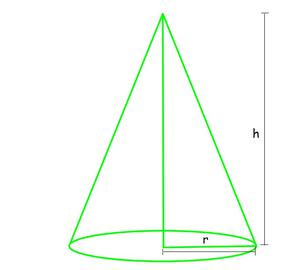### (ii) height 12 cm, slant height 13 cm

Solution:

Volume of cone (V) = (1/3) × πr2h

(i) Given values,

Radius of cone (r) = 7 cm

Slant height of cone (l) = 25 cm

h = √(l2 – r2)

h = √(252 – 72)

h = √576

h = 24 cm

V = (1/3) × (22/7) × 7 × 7 × 24                         (using π=22/7)

V = 22 × 7 × 8

V = 1232 cm3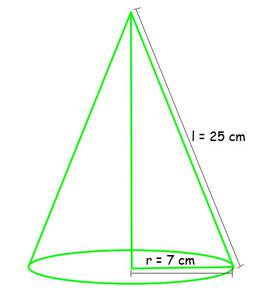(ii) Given values,

Height of cone (h) = 12 cm

Slant height of cone (l) = 13 cm

r = √(l2 – h2)

r = √(132 – 122)

r = √25

r = 5 cm

V = (1/3) × (22/7) × 5 × 5 × 12                            (using π=22/7)

V = 2200/7 cm3

V = 314.28 cm3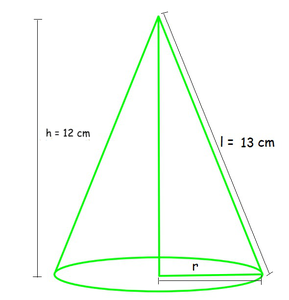### Question 3. The height of a cone is 15 cm. If its volume is 1570 cm3, find the radius of the base. (Use π = 3.14)

Solution:

Given values,

Height of cone (h) = 15 cm

Volume of cone (V) = 1570 cm3

V = (1/3) ×  πr2h

1570 = (1/3) × 3.14 × r2 × 15                         (using π=3.14)

r2 = 1570 × 3 / (3.14 × 15)

r2 = 100

r = √100

r = 10 cm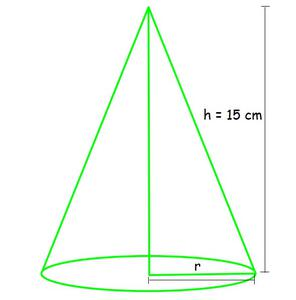### Question 4. If the volume of a right circular cone of height 9 cm is 48 π cm3, find the diameter of its base.

Solution:

Given values,

Height of cone (h) = 9 cm

Volume of cone (V) = 48π cm3

V = (1/3) × πr2h

48 × π = (1/3) × π × r2 × 9

r2 = 48 × 3 / 9          (canceling π from both sides)

r2 = 16

r = √16

r = 4 cm

Diameter = 2 times radius = 2 × r

= 2 × 4

= 8 cm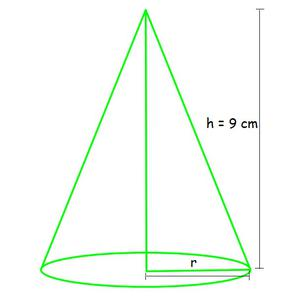### Question 5. A conical pit of top diameter 3.5 m is 12 m deep. What is its capacity in kiloliters?

Solution:

Given values,

Radius of cone (r) = 3.5/2 m

Height of cone (h) = 12 m

Volume of cone = (1/3) × πr2h

= (1/3) × (22/7) × (3.5/2) × (3.5/2) × 12                (taking π=22/7)

= (22 × 3.5 × 3.5 × 12) / (7 × 3 × 2 × 2)

= 38.5 m3

Capacity of conical pit in kilo liters:

1000 m3 = 1 liter

38.5 m3 = 1000 × 38.5 liters

= 38,500 liters

= 38.5 kilo liters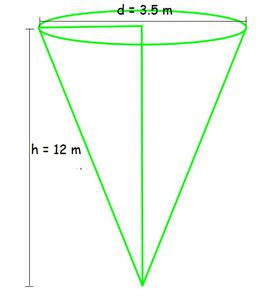### (iii) curved surface area of the cone

Solution:

Given values,

Radius of cone (r) = 28/2 = 14 cm

Volume of cone (V) = 9856 cm3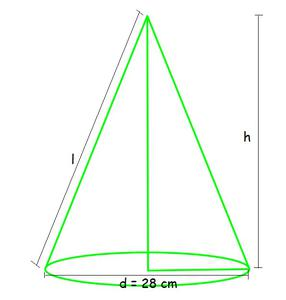(i) Volume of cone = (1/3) × πr2h

9856 = (1/3) × (22/7) × 14 × 14 × h                   (taking π=22/7)

h = (9856 × 3 × 7) / (22 × 14 × 14)

h = 48 cm

(ii) Let slant height = l

l2 = h2 + r2

l = √(h2 + r2)

l = √(482 + 142)

l = √(2304 + 196)

l = √2500

l = 50 cm

(iii) curved surface area of the cone = πrl

= π × 14 × 50 cm2

= 22/7 × 700                                   (taking π=22/7)

= 2,200 cm2

### Question 7. A right triangle ABC with sides 5 cm, 12 cm, and 13 cm is revolved about the side 12 cm. Find the volume of the solid so obtained.

Solution:

Here, after revolving the triangle about the side 12 cm, we get

Radius of cone (r) =5 cm

Height of cone (h) = 12 cm

Volume of cone = (1/3) × πr2h

= (1/3) × π × 5 × 5 × 12

= (12 × π × 5 × 5) / 3

V = 100π cm3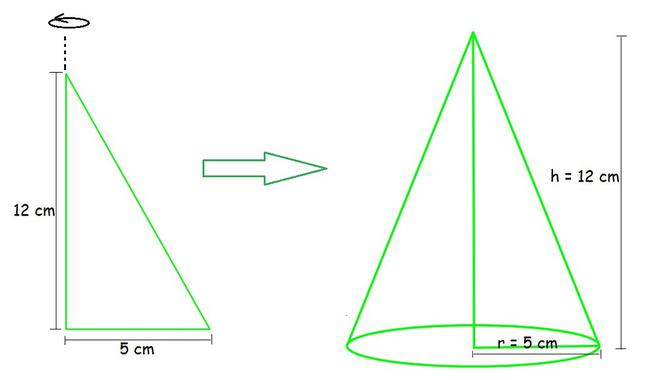### Question 8. If the triangle ABC in the Question 7 above is revolved about the side 5 cm, then find the volume of the solid so obtained. Find also the ratio of the volumes of the two solids obtained in Questions 7 and 8.

Solution:

Here, after revolving the triangle about the side 5 cm, we get

Radius of cone (r) =12 cm

Height of cone (h) = 5 cm

Volume of cone = (1/3) × πr2h

= (1/3) × π × 12 × 12 × 5

= (12 × π × 12 × 5) / 3

V = 240π cm3

Volume in Question 7 = 100π cm3

Ratio = (Volume in Question 8) / (Volume in Question 7)

= 240π/100π

= 12/5

Hence, the ratio obtained = 12 : 5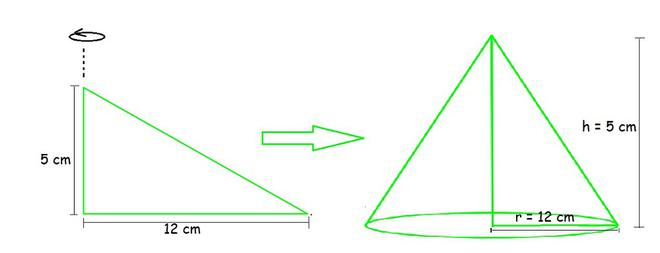### Question 9. A heap of wheat is in the form of a cone whose diameter is 10.5 m and height is 3 m. Find its volume. The heap is to be covered by canvas to protect it from rain. Find the area of the canvas required.

Solution:

Given values,

Radius of cone (r) = 10.5/2 = 105/20 m

Height of cone (h) = 3 m

Volume of cone = (1/3) × πr2h

= (1/3) × (22/7) × (105/2) × (105/2) × 3                (taking π=22/7)

= (22 × 105 × 105 × 3) / (3 × 20× 20× 7)

V = 86.625 m3

Area of the canvas = surface area of cone = πrl

Slant height (l) = √(h2 + r2)

l = √(32 + (10.5/2)2)

l = √(9+ (110.25/4))

l = √(146.25/4)

l = √36.56

l = 6.05 m (approx.)

Surface area of cone = π × (105/20) × 6.05 m2

= (22/7) × (635.25/20)                                         (taking π=22/7)

= 99.82 m2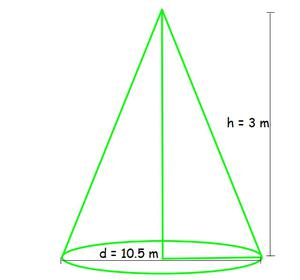Attention reader! All those who say programming isn’t for kids, just haven’t met the right mentors yet. Join the  Demo Class for First Step to Coding Coursespecifically designed for students of class 8 to 12.

The students will get to learn more about the world of programming in these free classes which will definitely help them in making a wise career choice in the future.

My Personal Notes arrow_drop_up